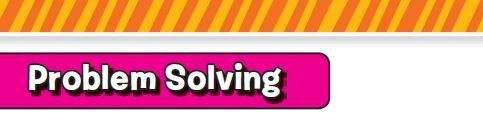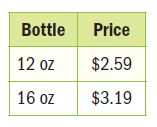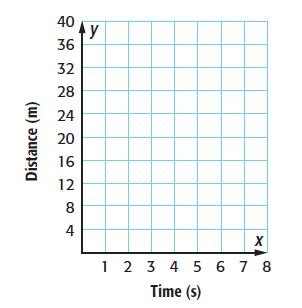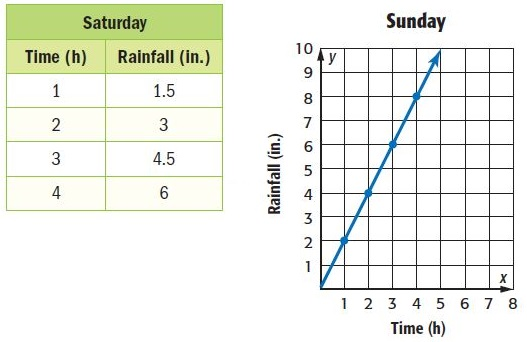Homework Explained - Math Practice 101Dear guest, you are not a registered member. As a guest, you only have read-only access to our books, tests and other practice materials.

As a registered member you can:

Registration is free and doesn't require any type of payment information. Click here to Register.
Go to page:• Question 1

Which bottle of shampoo shown at the right costs less per ounce?• $$\text{ The 16-ounces bottle}$$
• $$\text{The 12-ounces bottle}$$
• Question 2

An airplane is traveling at an average speed of 245 meters per second. How many kilometers per second is the plane traveling?

•  $$\text{km/s}$$
• Question 3

An Internet company charges $30 a month. There is also a$30 installation fee. Is the number of months you can have Internet proportional to the total cost? Explain.

• Question 4

Damon runs 8 meters in 1 second, 16 meters in 2 seconds, 24 meters in 3 seconds, and 32 meters in 4 seconds. Determine whether Damon’s distance is proportional to the number of seconds he runs by graphing on the coordinate grid at the right. Explain your reasoning.• Question 5

Suppose 3 televisions weigh 240.6 pounds. How much do 9 of the same televisions weigh?

•  $$\text{lb}$$
• Question 6

The table and the graph show the amount of rainfall for 2 different days. Which day had a greater rate of change? Explain.Yes, email page to my online tutor. (if you didn't add a tutor yet, you can add one here)